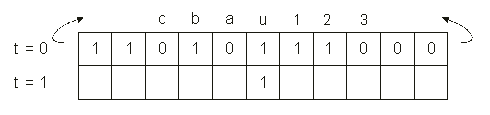GEP Book

Home
News
Author
Q&A
Tutorials
GEP Biblio
Contacts

Visit Gepsoft

 C. FERREIRA Complex Systems, 13 (2): 87-129, 2001

Gene Expression Programming: A New Adaptive Algorithm for Solving Problems

 The simplest CA is a wrap-around array of N binary-state cells, where each cell is connected to r neighbors from both sides. The state of each cell is updated by a defined rule. The rule is applied simultaneously in all the cells, and the process is iterated for t time steps. In the most frequently studied version of this problem, N = 149 and the neighborhood is 7 (the central cell is represented by “u”; the r = 3 cells to the left are represented by “c”, “b”, and “a”; the r = 3 cells to the right are represented by “1”, “2”, and “3”). Thus the size of the rule space to search for this problem is the huge number of 2128. Figure 14 shows a CA with N = 11 and the updated state for the cellular automaton “u” upon application of a certain transition rule.Figure 14. A one-dimensional, binary-state, r = 3 cellular automaton with N = 11. The arrows represent the periodic boundary conditions. The updated state is shown only for the central cell. The symbols used to represent the neighborhood are also shown. The task of density-classification consists of correctly determining whether ICs contain a majority of 1s or a majority of 0s, by making the system converge, respectively, to an all 1s state (black or “on” cells in a space-time diagram), and to a state of all 0s (white or “off” cells). Because the density of an IC is a function of N arguments, the actions of local cells with limited information and communication must be coordinated with one another to correctly classify the ICs. Indeed, to find rules that perform well is a challenge, and several algorithms were used to evolve better rules [14-17]. The best rules with performances of 86.0% (coevolution 2) and 85.1% (coevolution 1) were discovered using a coevolutionary approach between GA-evolved rules and ICs . However, the aim of this section is to compare the performance of GEP with GAs and GP when applied to a difficult problem. And, in fact, GEP does evolve better rules than the GP rule, using computational resources that are more than four orders of magnitude smaller than those used by GP.